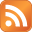# Script to calculate/show percentage of current year passed

Written by - 0 comments

Published on August 13th 2012 - Listed in Linux PHP Shell Coding

I was looking for a script which calculates the percentage of the current year passed. So for example on July 1st, the script's output would be around 50%.

On my quick research I haven't found a script, which gives me exactly this output, so I decided to do it myself.

As a shell/bash script:

#!/bin/bash
#########################################################################
# Script:       getpercentageofyear.sh                                  #
# Author:       Claudio Kuenzler www.claudiokuenzler.com                #
# Description:  Outputs percentage of year already passed               #
# History:      20120812 Script programmed                              #
#########################################################################
FIRST=\$(date --date="\$(date +%Y)-01-01 00:00:00" +%s)
LAST=\$(date --date="\$(date +%Y)-12-31 23:59:59" +%s)
NOW=\$(date +%s)

# Calculate how many seconds alone this whole year
RESYEAR=\$(( \$LAST - \$FIRST ))

# Calculate how many seconds this year so far
RESNOW=\$(( \$NOW - \$FIRST ))

# Calculate percentage
PERC=\$(( (\$RESNOW * 100) / \$RESYEAR ))

echo "\$PERC%"

exit 0

And as PHP script:

<?php
/////////////////////////////////////////////////////////////////////////
// Script:       getpercentageofyear.php                                /
// Author:       Claudio Kuenzler www.claudiokuenzler.com               /
// Description:  Outputs percentage of year already passed              /
// History:      20120812 Script programmed                             /
/////////////////////////////////////////////////////////////////////////
\$FIRST = mktime(00,00,00,01,01,date("Y"));
\$LAST = mktime(23,59,59,12,31,date("Y"));
\$NOW = time();

// Calculate how many seconds alone this whole year
\$RESYEAR = \$LAST - \$FIRST;

// Calculate how many seconds this year so far
\$RESNOW = \$NOW - \$FIRST;

// Calculate percentage and show 2 int after dot (XX.YY)
\$RES = substr((\$RESNOW*100)/\$RESYEAR,0,5);

echo "\$RES%";
?>

Who knows, it could become handy for someone else. Enjoy.

### Add a comment

Show form to leave a comment

### Comments (newest first)

No comments yet.